# C++ Sequential I/O Operations with Files

The file stream classes support a number of member functions for performing input and output operations on files. The functions get() and put() are capable of handling a single character at a time. The function getline() lets you handle multiple characters at a time. Another pair of functions, i.e., read() and write(), are capable of reading and writing blocks of binary data.

## The get(), getline(), and put() functions

The functions get() and put() are byte-oriented. That is, get() will read a byte of data, and put() will write a byte of data. The get() method has many forms, but the most commonly used version is shown here, along with put():

```istream &get(char &ch);
ostream &put(char ch);```

The get() function takes a single character as its input and reads it from the associated stream. It then stores the character's value in the variable ch. It provides a reference to the stream as its return value. The put() method returns a reference to the stream after it has been updated with the value of ch that was passed to it.

The following program brings up on the screen the contents of a selected file. The get() function is being used here.

```#include <iostream>
#include <fstream>
using namespace std;

int main()
{
char fname, ch;
ifstream fin;                 // create an input stream

cout << "Enter the name of the file: ";
cin.get(fname, 20);
cin.get(ch);

fin.open(fname, ios::in);     // open file
if (!fin)                     // if fin stores zero, i.e., a false value
{
cout << "An error occurred in opening the file.\n";
return 0;
}

while (fin)                   // When eof is reached, fin equals 0
{
cout << ch;                // Display the character
}

return 0;
}```

Let's suppose that the file "codescracker.txt" contains the following information: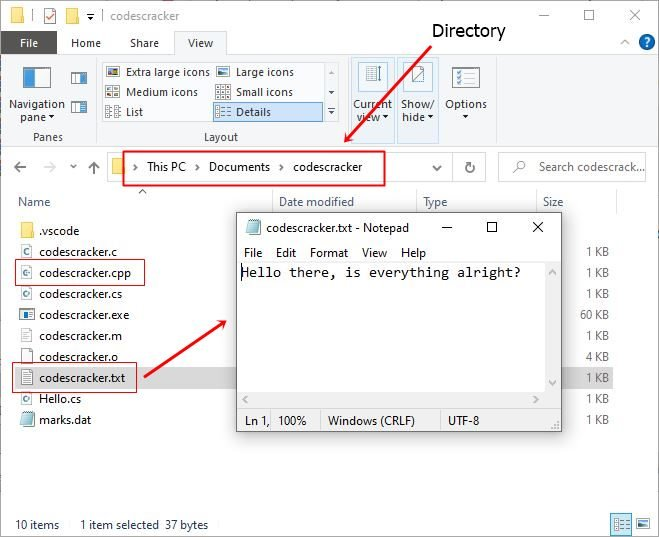As you can see, the file "codescracker.txt" is saved in the current directory, the directory where the above source code "codescracker.cpp" is saved. Now the following snapshot shows the initial output produced by the above program:Now enter the filename, say "codescracker.txt," and hit the ENTER key to produce the following output: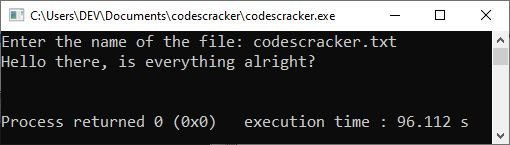As was previously mentioned, the stream that is associated with the file will become zero when the end of the file is reached. As a result, when "fin" reaches the end of the file, it will have a value of zero, which will cause the "while" loop to terminate.

The following piece of code makes use of the put() function in order to save all characters having an ASCII value between 33 and 127 in a text file with the name "aschars.txt."

```#include <iostream>
#include <fstream>
using namespace std;

int main()
{
ofstream fout;                  // create an output stream
char ch;
int line = 0, i;

fout.open("aschars.txt", ios::app);
if (!fout)                      // if fout is equal to zero
{
cout << "The file cannot be opened!\n";
return 0;
}

/* Write the characters */
for (i = 33; i < 128; i++)
fout.put((char)(i));

fout.close();

/* Now display the contents of the file. */
ifstream fin;
fin.open("aschars.txt", ios::in);
fin.seekg(0);
for (i = 33; i < 128; i++)
{
cout << i << " = ";         // Display the character
cout.put((char)(i));
cout << "\t";
if (!(i % 8))
{
cout << "\n";
line++;
}
if (line > 22)
{
system("PAUSE");
line = 0;
}
}

cout<<endl;
return 0;
}```

The following is a sample execution of the aforementioned C++ program: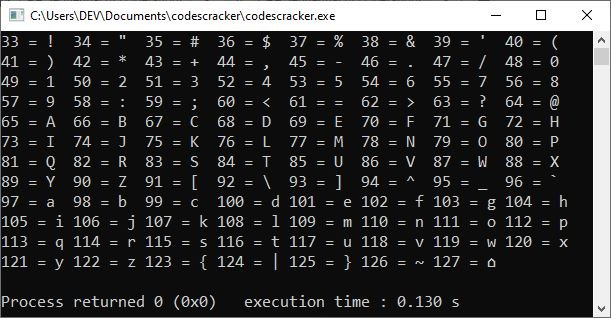### The Other Forms of the get() Function

In addition to the form shown earlier, there are several other forms of the get() function. The two most commonly used forms of the "get()" method are shown in the following two prototypes:

```istream & get(char ∗buf, int num, char delim = '\n');     // #1
int get();                                                // #2```

The first form (#1) begins to read characters into a character array that is pointed to by "buf" and continues to do so until either the "num" characters have been read or the "delim" character has been encountered, whichever comes first. As an illustration, consider the following statements:

```char line;
cin.get(line, 40, '\$');```

will continue reading characters into the "line" until either 40 characters have been read or a character with a dollar sign (whichever comes first) has been encountered. In other words, if the following information is provided in response to the statement made in the previous paragraph:

`Value is \$ 177.5`

"line" will then be storing

`Value is`

And if the given input is as follows:

`The amount is 17.5`

The contents of "line" will be

`The amount is 17.5`

The array pointed to by buf (or any other user-defined name) will be null-terminated by get(). If no delim character is specified, a newline character acts as a delimiter by default. If the delimiter character is encountered in the input stream, the get() function does not extract it. Rather, the delimiter character remains in the stream until the next input operation.

The second form (#2) of get() returns the next character from the stream. It returns EOF if the end of the file is encountered. For example, the following code fragment illustrates it:

```int i;
char ch;
ch = i = fin.get();```

If the input given is A, then the value of i will be 65 (the ASCII value of A), and the value of ch will be "A."

## The getline() function

getline() is yet another member function that accepts user input, and its prototype is as follows:

`istream &getline(char *buf, int num, char delim = '\n');`

As can be seen, this function is almost exactly the same as the version of get() that takes a buffer, a number, and a delimiter. In addition to that, the getline() function will read characters from the input stream and store them in the array that is referenced by the variable buf. This process will continue until either num characters have been read or the delimiter character that was specified is found. If the value of delim is not specified, the default behavior is for it to be a newline character.

## The read() and write() functions

Utilizing the read() and write() functions provided by C++ is an additional method for reading and writing blocks of binary data. Their models are as follows:

```istream &read((char *) &buf, int sizeof(buf));
ostream &write((char *) &buf, int sizeof(buf));```

The read() function reads bytes from the associated stream of sizeof(buf) (or any other integer value) and places them in the buffer pointed to by buf. The write() function writes sizeof(buf) (or any other integer value) bytes from the buffer pointed to by buf to the associated stream.

As you see, these functions take two arguments. The first is the address of variable buf, and the second is the length of that variable in bytes. The address of the variable must be type cast to type char * (i.e., a pointer to a character type).

The data written to a file using write() can only be read accurately using read().

Using the write() and read() functions, the following program writes a structure to disc and then reads it back in:

```#include <iostream>
#include <fstream>
#include <string>
using namespace std;

struct customer
{
string name;
float balance;
};

int main()
{
customer savac;
cout << "Put your name here: ";
getline(cin, savac.name);
cout << "Enter the balance: ";
cin >> savac.balance;

ofstream fout;
fout.open("Saving.txt", ios::out | ios::binary);
if (!fout)
{
cout << "Error opening the file!\n";
return 0;
}

fout.write((char *)&savac, sizeof(customer));
fout.close();

ifstream fin;
fin.open("Saving.txt", ios::in | ios::binary);
cout << "\n" << savac.name;
cout << " has a balance of \$" << savac.balance << " in his account.\n";
fin.close();

return 0;
}```

The following snapshot shows the sample run of the above program with the following user inputs: "William" as name and "430" as balance.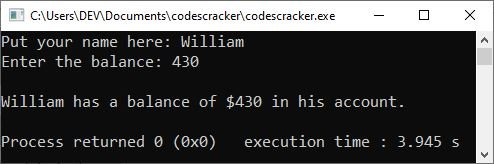It is clear that only a single invocation of the read() and write() functions is required to read or write the entirety of the structure. There is no requirement that each individual field be read or written in isolation.

If the end of the file is reached before the number of characters that have been specified has been read, read() will simply stop, and the buffer will contain the maximum number of characters that were available.

### Reading and Writing Class Objects

The functions read() and write() can also be used for reading and writing class objects. These functions handle the entire structure of an object as a single unit, using the computer's internal representation of data. For example, the function write() copies a class object from memory byte by byte with no conversion. But one thing that must be remembered is that only data members are written to the disc file and not the member functions.

The length of an object is obtained by the sizeof operator, and it represents the sum total of the lengths of all the data members of the object. Here is an example:

```#include <iostream>
#include <fstream>
using namespace std;

class student
{
char name;
float marks;

public:
void getdata(void);
void display(void);
};

void student::getdata(void)
{
char ch;
cin.get(ch);
cout << "Enter the name: ";
cin.getline(name, 20);
cout << "Enter the grade: ";
cout << "Enter the mark: ";
cin >> marks;
cout << "\n";
}

void student::display(void)
{
cout << "Name: " << name << "\tGrade: " << grade << "\tMarks: " << marks << "\n";
}

int main()
{
char fname;
student cse;        // declared an array of three objects
fstream fio;           // input and output files

cout << "Enter file name: ";
cin.get(fname, 20);
fio.open(fname, ios::in | ios::out);
if (!fio)
{
cout << "Error opening the file!\n";
return  0;
}

cout << "Enter details for the three students:\n\n";
for (int i = 0; i < 3; i++)
{
cse[i].getdata();
fio.write((char *)&cse[i], sizeof(cse[i]));
}

/* To access the file from the beginning,
* seekg(0) resets the file pointer to start.
*/
fio.seekg(0);

cout << "The contents of the " << fname << " file are shown below:\n";
for (int i = 0; i < 3; i++)
{
cse[i].display();
}
fio.close();

return 0;
}```

The following snapshot shows the sample run of the above program with user inputs: "codescracker.txt" as the name of the file and "William," "A," and "90" as the details for the first student; similarly, I supplied other details for the other two students.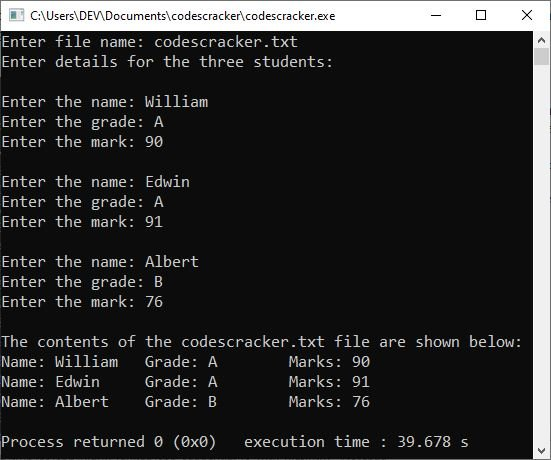#### More Examples

Here are some more C++ example programs listed, that you can go for:

C++ Quiz

« Previous Tutorial Next Tutorial »